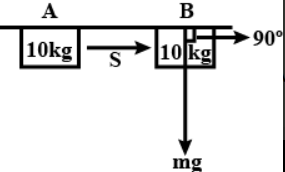Question

# A mass of is at a point on a table. It is moved to a point . If the line joining and is horizontal what is the work done on the object by the gravitational force? Explain your answer.

Medium
Updated on : 2022-09-05
SolutionVerified by Toppr

## Hint: Observe the angle between force of gravity and displacement. Explanation: Step 1: Concept used:Work done is given by:where, force applied displacement angle between the force applied and displacement. Step 2: Finding the work done:In the given problem, the force of gravity and displacement are perpendicular to each other.Hence, the work done is zero.

Video Explanation00